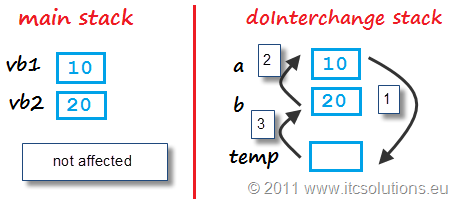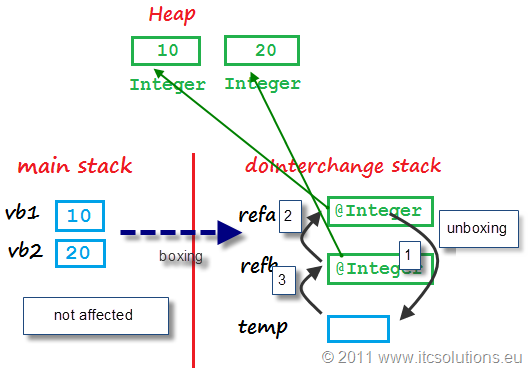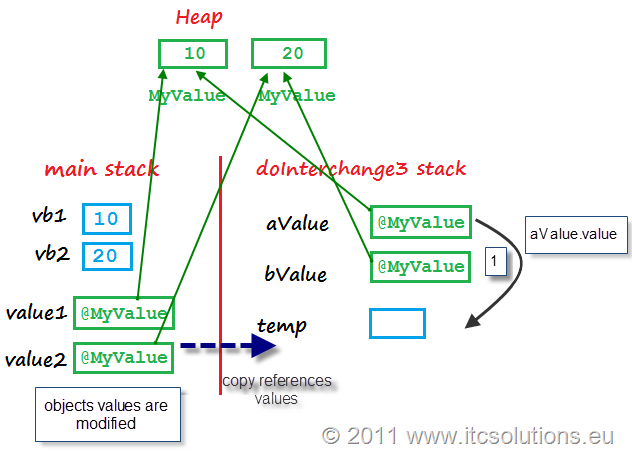# Tutorial Java SCJP – #14 Methods and passing variables into methods

In Java, methods define the object behavior or implement different functionalities at class level (for static methods). If variables (value type or references) represent the static part of Java programming,then methods represent the dynamic part because methods are equivalent to code blocks that are executed and that are processing some variables.

Other topics that are part of this Java tutorial are accessible through Java 6 Tutorial – Contents.

## How to define methods

The general syntax used to define methods is

[access_modifier] [non-access modifier] return_type method_name (type parameter1, type parameter2, …, type parameterN)

where (access modifiers and non-access ones will be described better in a separate topic):

access_modifier – define the visibility of the method; possible values are:

• default – when you don’t write anything;
• public – methods is visible in other classes;
• private – methods are not visible from other classes;
• protected – methods are visible only in subclasses.

non-access modifier – define other attributed for the method like: static (the method is defined at class level – it’s not an object method), final (the method can’t be overridden in subclasses), abstract (the method can’t be instantiated because contains abstract methods – virtual pure methods)

return_type – any primitive or reference type; if the method doesn’t return a value then the return type is void; if you define a return type, then the method must end with a return value/variable statement, where the value/variable type is the defined return type:

```public class Test {
public static void main(String[] args) {
int vb1 = 10;
int vb2 = 20;
//calling the doSum method
int sum = doSum(vb1, vb2);
System.out.println("The sum is "+sum);
}
public static int doSum(int a, int b)
{
int result = a + b;
return result;
}
}```

method_name – must respect the same naming convention used for variables; Java recommends using camelCase naming convention (the first word starts with a small letter); also, the names should be verb-noun pairs, like getName, doSum;

parameters – represent the input values (primitive or references) for the method; regarding parameters you must know this:

• the method header variables are called parameters; when you call a method, the input values are called arguments;
• methods input parameters are local variables for the method;
• you can define a variable number of input parameters – var-arg methods;

One important rule regarding parameters is:

• method arguments are always passed by their value; methods parameters are always copies of the arguments (maybe the most important rule);
• a primitive parameter represent a value copy of the calling argument;
• a reference parameter represent a value copy (the reference value IS NOT AN OBJECT, but the OBJECT ADDRESS) of the calling reference argument;

If you remember also rules regarding Tutorial Java – #8 Understand Stack and Heap, then the next example

```class MyValue
{
public int value;
}
public class Main {
public static void main(String[] args) {
int vb1 = 10;
int vb2 = 20;
//calling the doSum method
int sum1 = doSum(vb1, vb2);
System.out.println("The sum is "+sum1);

MyValue ref1 = new MyValue();
MyValue ref2 = new MyValue();
ref1.value = 100;
ref2.value = 200;

//calling the doObjectSum method
int sum2 = doObjectSum(ref1, ref2);
System.out.println("The objects sum is "+sum2);
}
//the method parameters are 2 integer values
public static int doSum(int a, int b)
{
int result = a + b;
return result;
}
//the method parameters are 2 references
public static int doObjectSum(MyValue v1, MyValue v2)
{
int result = v1.value + v2.value;
return result;
}
}```

generates in memory a image like this:

From the previous image, you can see that the methods arguments are values of the calling variables.

## How to pass parameters and return multiple values

Before we describe this concept, keep in mind one important Java rule: Method arguments are always passed by their value; methods parameters are always copies of the arguments.

There are 2 types of parameters: value type and object references. The difference between them is a logical one because references are like numerical variables with an important characteristic: their values represent addresses of objects stored in Heap.

In C++ things are different because we have arguments passed by their value and also by their address (when you use pointers). Also, in C# you have arguments passed by their values or by their pointers (unsafe) or using ref as input parameters attribute.

Because , in Java, method arguments are always passed by their values, it means that method local variables have the same value as the calling arguments. This may represent a problem when we want to write methods that return more than one value (for one value, you can use the method return type).

For example, let’s write a method used to interchange the values of 2 primitive variables. If we write like this:

```public class Main {
public static void main(String[] args) {
int vb1 = 10;
int vb2 = 20;
doInterchange(vb1, vb2);
System.out.println("vb1 = "+vb1+", vb2 = "+vb2);
}
public static void doInterchange(int a, int b)
{
int temp = a;
a = b;
b = temp;
}
}```

then the output is

`vb1 = 10, vb2 = 20`

Despite the doInterchange() method is implementing the correct algorithm, the output is not the expected one because, based on the previous rule, the method is interchanging the values of its own local variables a and b.So we must write a better method, that will interchange the values of 2 main variables. Let’s try with references by boxing the 2 integers into their corresponding objects, like this:

```public class Main {
public static void main(String[] args) {
int vb1 = 10;
int vb2 = 20;
doInterchange2(vb1, vb2);	//the values are boxed by default
System.out.println("vb1 = "+vb1+", vb2 = "+vb2);
}
public static void doInterchange2(Integer ra, Integer rb)
{
int temp = ra;	//default unboxing
ra = rb;
rb = temp;	//boxing
}
}```

but, surprise, the output is the same

`vb1 = 10, vb2 = 20`Interchange with Integer references - doInterchange2

In this case, the reason is more subtle because the default boxing mechanism is creating a new object with the value of the primitive variable. Also keep in mind that Integer objects are immutable (see Tutorial Java SCJP – #12 Immutable, String and Integer).

The correct solution for the interchange method is to use references of your own classes, like this:

```class MyValue
{
public int value;

public MyValue(int input)
{
value = input;
}
}
public class Main {
public static void main(String[] args) {
int vb1 = 10;
int vb2 = 20;

MyValue value1 = new MyValue(vb1);
MyValue value2 = new MyValue(vb2);
doInterchange3(value1, value2);
vb1 = value1.value;
vb2 = value2.value;
System.out.println("vb1 = "+vb1+", vb2 = "+vb2);
}

public static void doInterchange3(MyValue aValue, MyValue bValue)
{
int temp = aValue.value;
aValue.value = bValue.value;
bValue.value = temp;
}
}```

The previous example generates the desired output

`vb1 = 20, vb2 = 10`

because the references from main stack and from doInterchange3 stack reference the same objects in Heap:Interchange with objects references - Interchange3

Because, in Java, method arguments are always passed by their values (also references) DO NOT make the mistake to think this will work:

```    public static void doInterchange4(MyValue aValue, MyValue bValue)
{
//interchange references VALUES and NOT objects values
MyValue temp = aValue;
aValue = bValue;
bValue = temp;
}```

Remember, aValue reference value is the copy of the value1 reference value.

Another solution, is to use an array as a wrapper for the primitive values, like this:

```public class Main {
public static void main(String[] args) {
int vb1 = 10;
int vb2 = 20;

int[] anArray = {vb1,vb2};
doInterchange5(anArray);
vb1 = anArray;
vb2 = anArray;
System.out.println("vb1 = "+vb1+", vb2 = "+vb2);
}

public static void doInterchange5(int[] values)
{
int temp = values;
values = values;
values = temp;
}
}```

Regarding how to pass variables into methods in Java you must also read:

## What you must remember as important facts for the SCJP certification exam:

Methods input parameters are represented by primitive and/or references;

When you define a method, the header variables are called parameters;

When you call a method the input values are called arguments;

Methods input parameters are local variables for the method;

Method arguments are always passed by their value; methods parameters are always copies of the arguments (maybe the most important rule);

A primitive parameter represent a value copy of the calling argument;

A reference parameter represent a value copy (the reference value IS NOT AN OBJECT, but the OBJECT ADDRESS) of the calling reference argument;

Shadowing  occurs when the input parameters has the same name as a local variable or instance variable (very difficult to debug because it is a logical error); for instance variables use this. notation to distinguish between input parameter and the object attribute; for class static variables use class name;

Methods can have variable number arguments (var-args methods);

A var-arg method can have only one var-arg parameter; the var-arg must be the last parameter (if the method has other normal parameters);

Other topics that are part of this Java tutorial are accessible through Java 6 Tutorial – Contents.